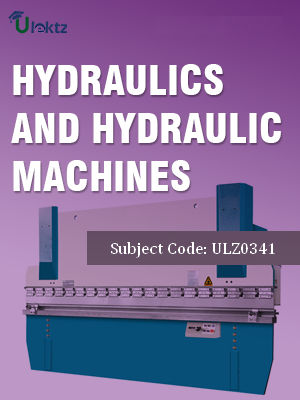•My WalletMy Order
•My Profile
•My Connections
•My Books
•My Videos
•My Tests
•My Calender
•My Messages
•My Shopping Cart
•My Orders
•Account Settings
•Help

# Book Details# Hydraulics And Hydraulic Machines

 Course Code : ULZ0341 Author : uLektz University : General for All University Regulation : 2017 Categories : Civil Format :ePUB3 (DRM Protected) Type : eBook

FREE

Description :Hydraulics And Hydraulic Machines of ULZ0341 covers the latest syllabus prescribed by General for All University for regulation 2017. Author: uLektz, Published by uLektz Learning Solutions Private Limited.

Note : No printed book. Only ebook. Access eBook using uLektz apps for Android, iOS and Windows Desktop PC.

##### Topics
###### UNIT-1 DIMENSIONAL ANALYSIS AND MODEL STUDIES

1.1 Introduction, Systems of units, Dimensions of quantities, Dimensional Homogeneity of an equation.

1.2 Analysis- Raleigh’s method, Buckingham’s Π theorem- problems.

1.3 Model Studies, Similitude, Non-dimensional numbers: Froude model, Undistorted and Distorted models, Reynold’s models- Problems

###### UNIT-2 UNIFORM FLOW IN OPEN CHANNELS

2.1 Introduction, Geometric properties of Rectangular, Triangular, Trapezoidal and Circular channels.

2.2 Chezy’s equation, Manning’s equation-problems.

2.3 Most economical open channels, Rectangular, Triangular, Trapezoidal and Circular channeles- problems.

###### UNIT-3 NON-UNIFORM FLOW IN OPEN CHANNELS

3.1 Introduction, Specific energy, Specific energy diagram, Critical depth, Conditions for Critical flow- Theory & problems.

3.2 Hydraulic jump in a Horizontal Rectangular Channel- Theory and problems.

3.3 Dynamic equation for Non-Uniform flow in an Open channel, Classification of Surface profiles- simple Problems.

###### UNIT-4 IMPACT OF JET ON FLAT VANES

4.1 Introduction, Impulse- Momentum equation.

4.2 Direct impact of a jet on a stationary flat plate, Oblique impact of a jet on a stationary flat plate, Direct impact on a moving plate, Direct impact of a jet on a series of a jet on a series of flat vanes on a wheel.

4.3 Conditions for maximum hydraulic efficiency, Impact of a jet on a hinged flat plate- problems.

###### UNIT-5 IMPACT OF JET ON CURVED VANES

5.1 Introduction, Force exerted by a jet on a fixed curved vane, moving curved vane.

5.2 Introduction to concept of velocity triangles, Impact of jet on a series of curved vanes-problems.

###### UNIT-6 PELTON WHEEL

6.1 Introduction to Turbines, Classification of Turbines.

6.2 Pelton wheel- components, working and velocity triangles, Maximum power, efficiency, working proportions- problems.

###### UNIT-7 KAPLAN TURBINES

7.1 Introduction, Components, Working and Velocity triangles, Properties of the Turbine, Discharge of the Turbines, Number of Blades-Problems.

7.2 Draft Tube: Types, efficiency of a Draft tube, Introduction to Cavitation in Turbines.

###### UNIT-8 CENTRIFUGAL PUMPS

8.1 Introduction, Classification, Priming, methods of priming.

8.2 Heads and Efficiencies, Equation for work done, minimum starting speed, velocity triangles.

8.3 Multistage Centrifugal Pumps ( Pumps in Series and Pumps in parallel), Characteristic Curves for a Single stage Centrifugal Pumps- problems.

### Related Books